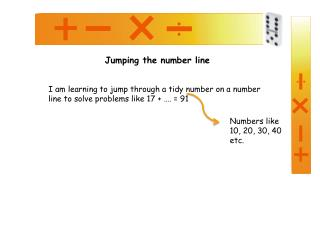DownloadDownload PresentationJumping the number line

# Jumping the number line

Télécharger la présentation## Jumping the number line

- - - - - - - - - - - - - - - - - - - - - - - - - - - E N D - - - - - - - - - - - - - - - - - - - - - - - - - - -
##### Presentation Transcript

1. Jumping the number line I am learning to jump through a tidy number on a number line to solve problems like 17 + …. = 91 Numbers like 10, 20, 30, 40 etc.

2. SAMPLE PROBLEM Craig works on the 18th floor of a building and wants to visit his friend who lives on the 72nd floor of the building. How many levels must be climb through? Friend: 72nd floor Craig: 18th floor 18 + ? = 72

3. SAMPLE PROBLEM 18 + ? = 72 Step 1: Draw a number line with your starting point (that’s the level where Craig is working.) Friend: 72nd floor Step 2: Write in the end point (that’s the level where Craig’s friend is working.) Craig: 18th floor 18 72

4. SAMPLE PROBLEM 18 + ? = 72 Step 3: Choose a tidy number to jump up to which takes you just above your target then write it on your number line. 30 is a tidy number, but it’s not the closest one 20 is perfect. +2 18 20 72

5. SAMPLE PROBLEM 18 + ? = 72 Step 4: Now I think 20 + ? = 72 I know this is 52 so I add this arrow to my diagram. +52 +2 18 20 72

6. SAMPLE PROBLEM 18 + ? = 72 Working out the answer: You jumped up 2 then up 52 (2 + 52 = 54). 18 + 54 = 72 Friend: 72nd floor +54 Craig: 18th floor

7. Now let’s try one together Sarah works on floor 27 of the office block and wants to meet her friend who works on the 62nd floor. How many floors does she need to climb? 27 + ? = 62

8. Now let’s try one together 27 + ? = 62 Step 1: Draw a number line with your starting point and end point. Do this now on a piece of paper.

9. Now let’s try one together 27 + ? = 62 Step 2: Choose a tidy number to jump up to which takes you just past your target number (27). Do this now.

10. Now let’s try one together 27 + ? = 62 Step 3: Now find the number you must jump up to get to the end of your number line (62). Add this to your drawing now.

11. Now let’s try one together 15 + ? = 61 Step 4: Now work out how far you had to jump. (Add your first jump and your second jump together.) Do this now. So… 15 + 35 = 61

12. Now try these questions on your own. • 18 + ….. = 54 • 27 + ….. = 62 • 19 + … = 96 • 38 + …. = 81 • 37 + ….. = 56 6) 17 + ….. = 36 7) 48 + …. = 94 8) 39 + ….. = 63 9) 28 + …. = 73 10) 58 + …. = 97

13. Only click again if you have completed all the questions on the previous slide and you are ready to see the answers. • 36 • 35 • 77 • 43 • 19 6) 19 7) 46 8) 24 9) 45 10) 39 Need some more practise? Try Pg44 of Teacher Tools Addition and Subtraction Book Numeracy resources